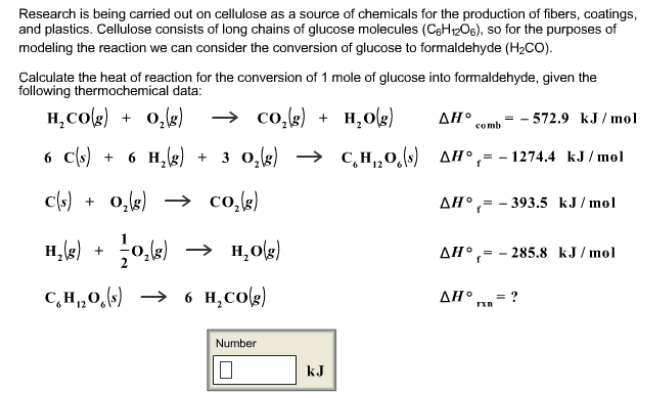Chemistry Practice Problems Hess's Law Practice Problems Solution: Research is being carried out on cellulose as a so...

🤓 Based on our data, we think this question is relevant for Professor Sevy's class at BYU.

# Solution: Research is being carried out on cellulose as a source of chemicals for the production of fibers, coatings and plastics. Cellulose consists of long chains of glucose molecules (C6H12O6), so for the purposes of modeling the reaction we can consider the conversion of glucose to formaldehyde (H2CO). Calculate the heat of reaction for the conversion of 1 mole of glucose into formaldehyde, given the following thermochemical data:H2CO (g) + O2 (g) → CO2 (g) + H2O (g)     ΔH°comb = -572.9 kJ/mol 6C (s) + 6H2 (g) + 3O2 (g) → C6H12O6 (s)     ΔH°f = -1274.4 kJ/mol C (s) + O2 (g) → CO2 (g)     ΔH°f = -393.5 kJ/mol H2 (g) + 1/2O2 (g) → H2O (g)     ΔH°f = -285.8 kJ/mol C6H12O6 (s) → 6H2CO (g)     ΔH°rxn = ?

###### Problem

Research is being carried out on cellulose as a source of chemicals for the production of fibers, coatings and plastics. Cellulose consists of long chains of glucose molecules (C6H12O6), so for the purposes of modeling the reaction we can consider the conversion of glucose to formaldehyde (H2CO).

Calculate the heat of reaction for the conversion of 1 mole of glucose into formaldehyde, given the following thermochemical data:

H2CO (g) + O2 (g) → CO(g) + H2O (g)     ΔH°comb = -572.9 kJ/mol

6C (s) + 6H2 (g) + 3O2 (g) → C6H12O(s)     ΔH°f = -1274.4 kJ/mol

C (s) + O2 (g) → CO2 (g)     ΔH°f = -393.5 kJ/mol

H2 (g) + 1/2O2 (g) → H2O (g)     ΔH°f = -285.8 kJ/mol

C6H12O6 (s) → 6H2CO (g)     ΔH°rxn = ?View Complete Written Solution

Hess's Law

Hess's Law

#### Q. Enter your answer in the provided box.From these data, S (rhombic) + O2 (g) → SO2 (g)            ΔH°rxn = -296.06 kJ/mol S (monoclinic) + O2 (g) → SO2...

Solved • Thu Aug 09 2018 12:53:36 GMT-0400 (EDT)

Hess's Law

#### Q. Given that H2 (g) + F2 (g) → 2HF (g)     ΔH°rxn = - 546.6 kJ 2H2 (g) + O2 (g) → 2H2O (l)     ΔH°rxn = - 571.6 kJ calculate the value of ΔH°rxn for 2F2...

Solved • Wed Aug 08 2018 12:38:43 GMT-0400 (EDT)

Hess's Law

#### Q. Given these reactions, X (s) + 1/2O2 (g) → XO (s)     ΔH = -993.7 kJ XCO3 (s) → XO (s) + CO2 (g)     ΔH = +424.5 kJ What is ΔHrxn for this reaction? X...

Solved • Wed Aug 08 2018 12:35:17 GMT-0400 (EDT)

Hess's Law

#### Q. Calculate ΔH°rxn for the reaction 2Ni (s) + 2S (s) + 3O2 (g) → 2NiSO3 (s) from the following information: (1) NiSO3 (s) → NiO (s) + SO3 (g)     ΔH°rxn...

Solved • Wed Aug 08 2018 12:17:35 GMT-0400 (EDT)